## Chess and Recursion: Part 1

I’ve been using my investment time at thoughtbot to build a multiplayer chess game using Elixir and Phoenix in order to hone my skills in that area. One of the trickiest and most fun parts of the project so far has been generating all the possible moves for a player to make.

### The board

We will store the board as a map of pieces indexed by their position as a tuple. This makes it easy to move pieces around the board by popping elements out of the map and then adding them back in with a new index.

``````%{
{0, 7} => %{type: "rook",   colour: "black"},
{1, 7} => %{type: "knight", colour: "black"},
{2, 7} => %{type: "bishop", colour: "black"},
{3, 7} => %{type: "queen",  colour: "black"},
{4, 7} => %{type: "king",   colour: "black"},
{5, 7} => %{type: "bishop", colour: "black"},
{6, 7} => %{type: "knight", colour: "black"},
{7, 7} => %{type: "rook",   colour: "black"},

{0, 6} => %{type: "pawn", colour: "black"},
{1, 6} => %{type: "pawn", colour: "black"},
# rest of the pieces ...
}
``````

### Linear moves

We’ll start this journey with rooks as they have a relatively straightforward movement profile. For now we will ignore blocking pieces which means that for each direction we just need to traverse the board in one direction until we hit the edge.

Looping in Elixir is achieved through recursion. This may sound complex but has some advantages as we will see.

Here’s a function that will return all the available moves in one direction:

``````# lib/chess/moves/rook.ex

defmodule Chess.Moves.Rook do
def moves_north(_board, {_file, 7}), do: []

def moves_north(board, {file, rank}) do
[{file, rank + 1} | moves_north(board, {file, rank + 1})]
end
end
``````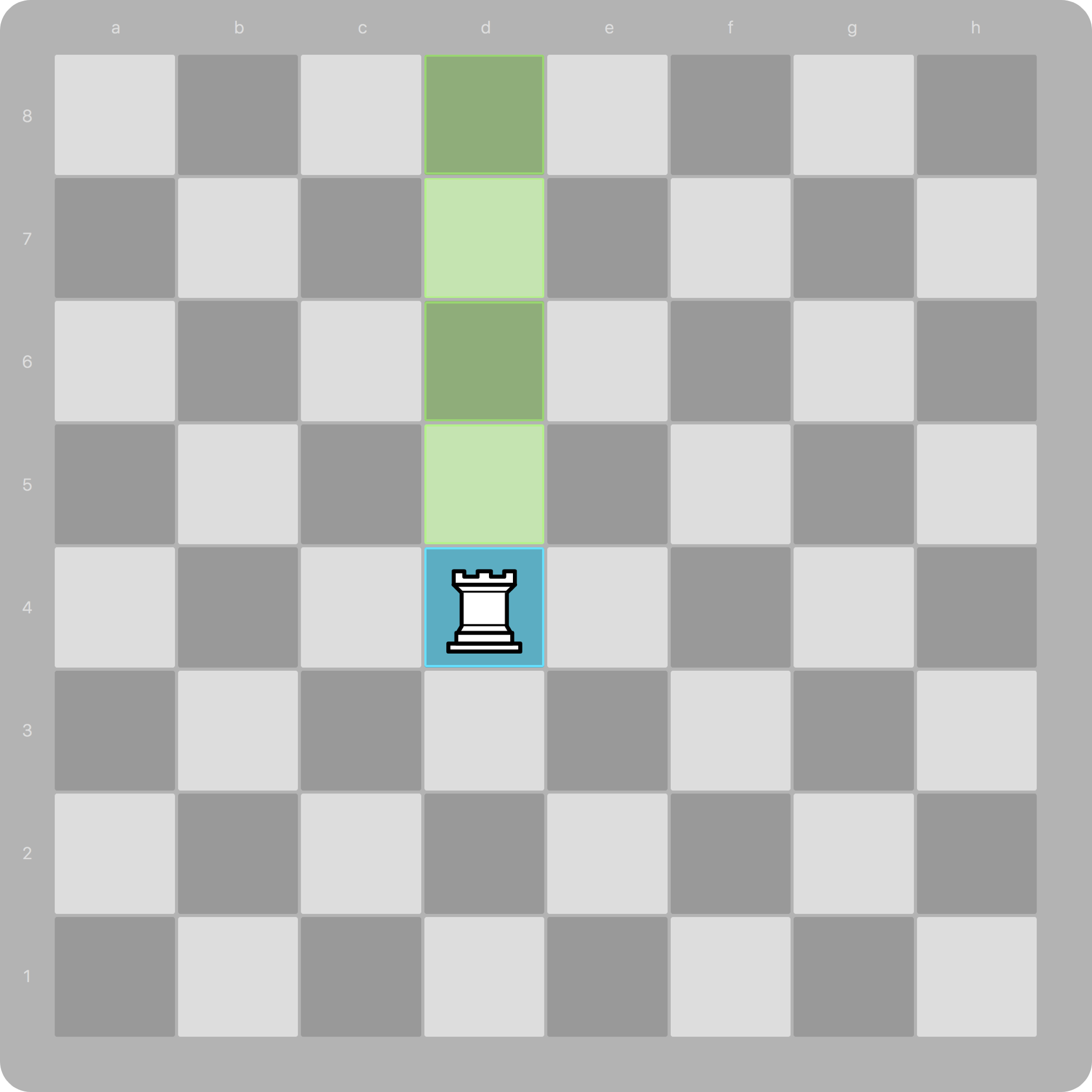Let’s break this down piece by piece.

We’re using Elixir’s pattern matching to define multiple functions here with the same name. If you’d like to read more about Elixir’s pattern matching I would recommend Pattern Matching in Elixir: Five Things to Remember by Anna Neyzberg

The first function matches if the `rank` is 7. This means we’ve hit the top edge of the board so we return an empty list to stop the recursion.

``````def moves_north(_board, {_file, 7}), do: []
``````

(The underscores in front of the variable names indicate that we can discard the values as we don’t need them in the function definition.)

Lists in Elixir are represented internally as linked lists. They are represented internally by pairs consisting of the head and the tail of the list. The `|` operator allows us to match the head and tail of a lists or construct a new list from a head and a tail.

The next function returns a new list with the head containing the next square north and the tail being the result of another call to this function:

``````def moves_north(board, {file, rank}) do
[{file, rank + 1} | moves_north(board, {file, rank + 1})]
end
``````

Essentially we are adding `1` to the rank until it reaches `7`, then unwinding the stack and returning the resulting list. If `rank` starts at say `4` (we’ll set `file` to `0`) then we get this back from the recursive function:

``````[{0, 4} | [{0, 5} | [{0, 6} | [{0, 7}]]]]
``````

Which is equivalent to:

``````[{0, 4}, {0, 5}, {0, 6}, {0, 7}]
``````

And there we have our list of moves in one direction!

We can create other recursive functions to handle moving south, east and west:

``````def moves_south(_board, {_file, 0}), do: []
def moves_south(board, {file, rank}) do
[{file, rank - 1} | moves_south(board, {file, rank - 1})]
end

def moves_east(_board, {7, _rank}), do: []
def moves_east(board, {file, rank}) do
[{file + 1, rank} | moves_east(board, {file + 1, rank})]
end

def moves_west(_board, {0, _rank}), do: []
def moves_west(board, {file, rank}) do
[{file - 1, rank} | moves_west(board, {file - 1, rank})]
end
``````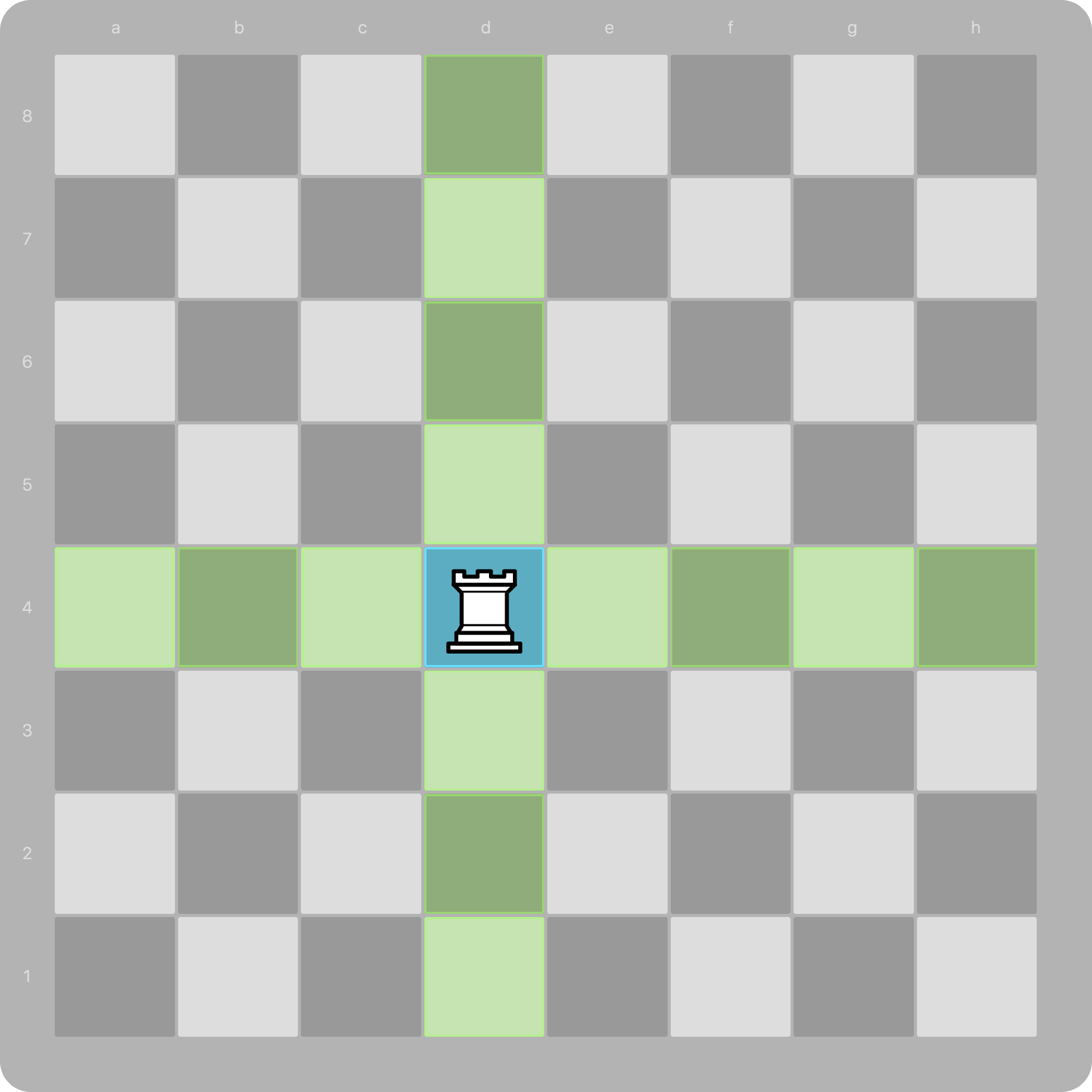We can even start writing functions to handle moving in diagonal directions, for bishops and queens:

``````def moves_northeast(_board, {7, _rank}), do: []
def moves_northeast(_board, {_file, 7}), do: []
def moves_northeast(board, {file, rank}) do
[{file + 1, rank + 1} | moves_northeast(board, {file + 1, rank + 1})]
end
``````

We’re writing a lot of functions now that look very similar, so let’s figure out a better way.

Each of these functions is doing the same thing—moving in a straight line—just in a different direction. We could pass an offset vector as a tuple instead of hard coding it into each function, so if we want all the moves north we could call it like this:

``````moves(board, {3, 4}, {0, 1}) # (board, position, vector)
``````

The main body of the function looks like this:

``````def moves(board, {file, rank}, {fv, rv}) do
next_square = {file + fv, rank + rv}
[next_square | moves(board, next_square, {fv, rv})]
end
``````

We need to match cases where we hit the edge of the board and stop the recursion:

``````def moves(_board, {0, _rank}, {-1, _}), do: []
def moves(_board, {_file, 0}, {_, -1}), do: []
def moves(_board, {7, _rank}, {1, _}), do: []
def moves(_board, {_file, 7}, {_, 1}), do: []

def moves(board, {file, rank}, {fv, rv}) do
next_square = {file + fv, rank + rv}
[next_square | moves(board, next_square, {fv, rv})]
end
``````

### Obstructions

We need to handle cases where another piece is in the way. First, let’s define a function that will tell us if a square is empty:

``````# we'll use defp to define a private function as
# we won't be calling this outside of this module.
defp empty?(board, position) do
is_nil(board[position])
end
``````

Now we can use this function in our moves function to only recurse if the next square is empty. If the square is not empty then we return an empty list to stop recursion.

``````def moves(board, {file, rank}, {fv, rv}) do
next_square = {file + fv, rank + rv}
if empty?(board, next_square) do
[next_square | moves(board, next_square, {fv, rv})]
else
[]
end
end
``````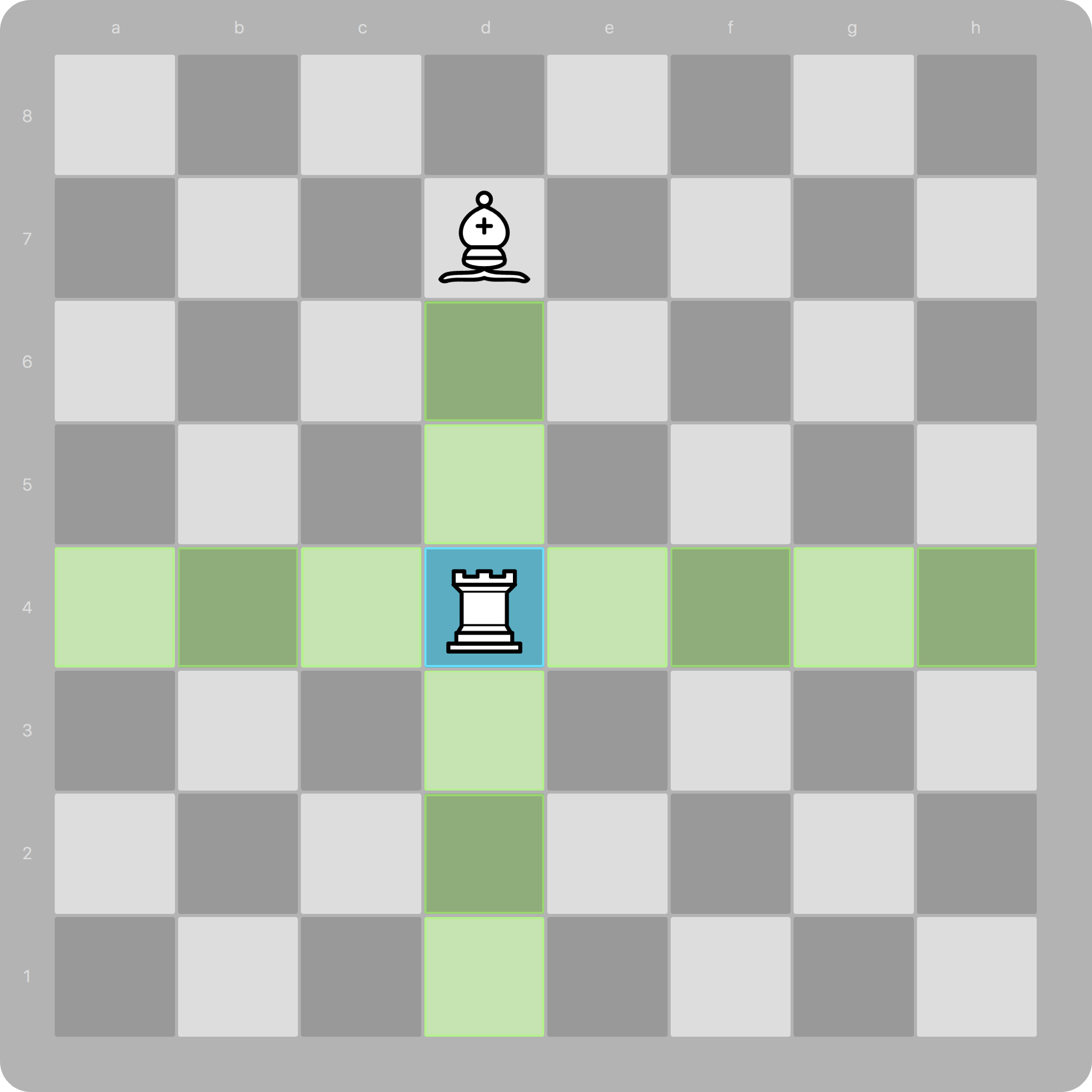### All together now

Lastly, let’s combine these to generate moves for all the pieces that move in straight lines:

``````defmodule Chess.Moves do
def queen(board, position) do
# The queen moves like both a rook and a bishop
rook(board, position) ++
bishop(board, position)
end

def rook(board, {file, rank}) do
moves(board, {file, rank}, {0, 1}) ++
moves(board, {file, rank}, {0, -1}) ++
moves(board, {file, rank}, {-1, 0}) ++
moves(board, {file, rank}, {1, 0})
end

def bishop(board, {file, rank}) do
moves(board, {file, rank}, {1, 1}) ++
moves(board, {file, rank}, {1, -1}) ++
moves(board, {file, rank}, {-1, 1}) ++
moves(board, {file, rank}, {-1, -1})
end

defp moves(_board, {0, _rank}, {-1, _}), do: []
defp moves(_board, {_file, 0}, {_, -1}), do: []
defp moves(_board, {7, _rank}, {1, _}), do: []
defp moves(_board, {_file, 7}, {_, 1}), do: []
defp moves(board, {file, rank}, {fv, rv}) do
next_square = {file + fv, rank + rv}
if empty?(board, next_square) do
[next_square | moves(board, next_square, {fv, rv})]
else
[]
end
end

defp empty?(board, position) do
is_nil(board[position])
end
end
``````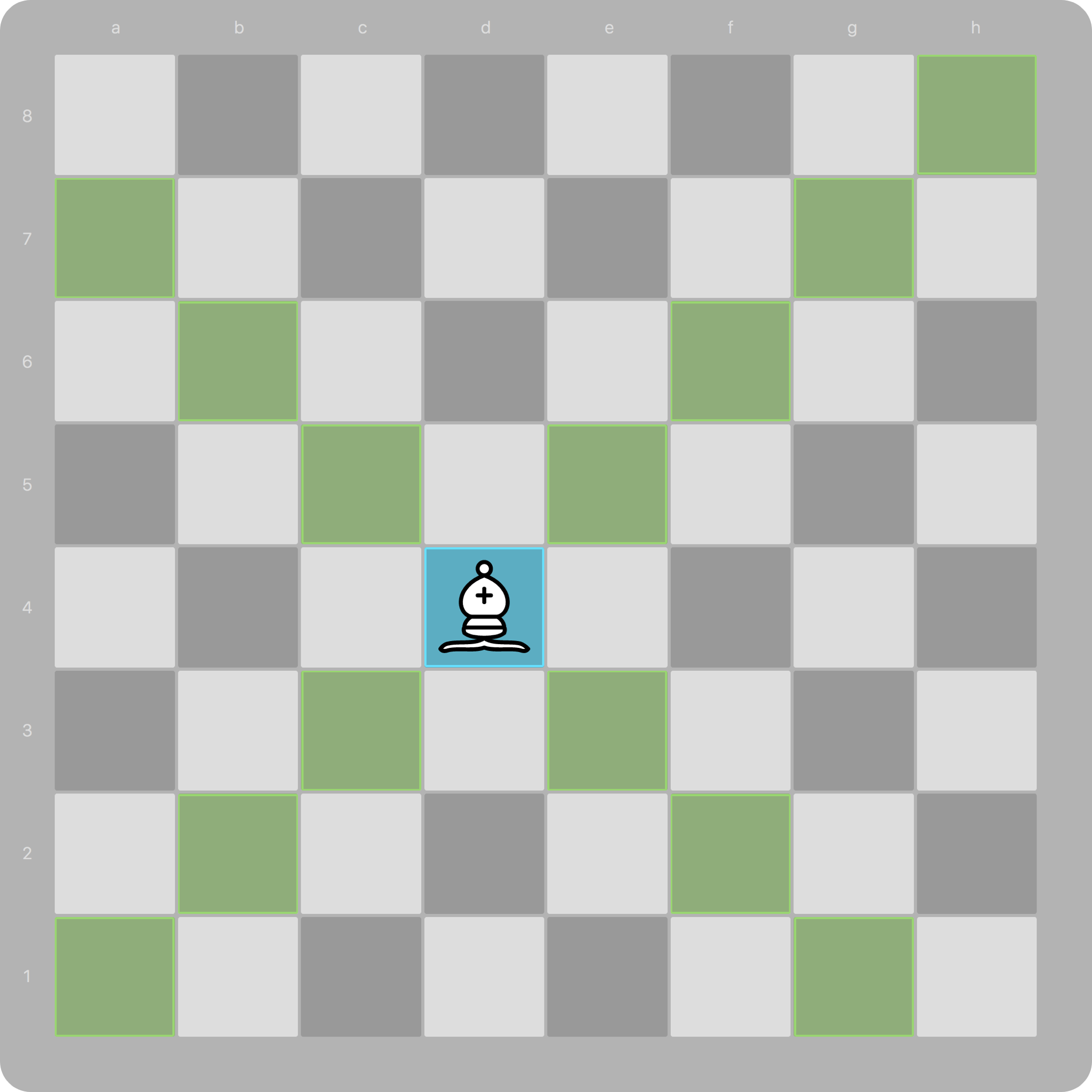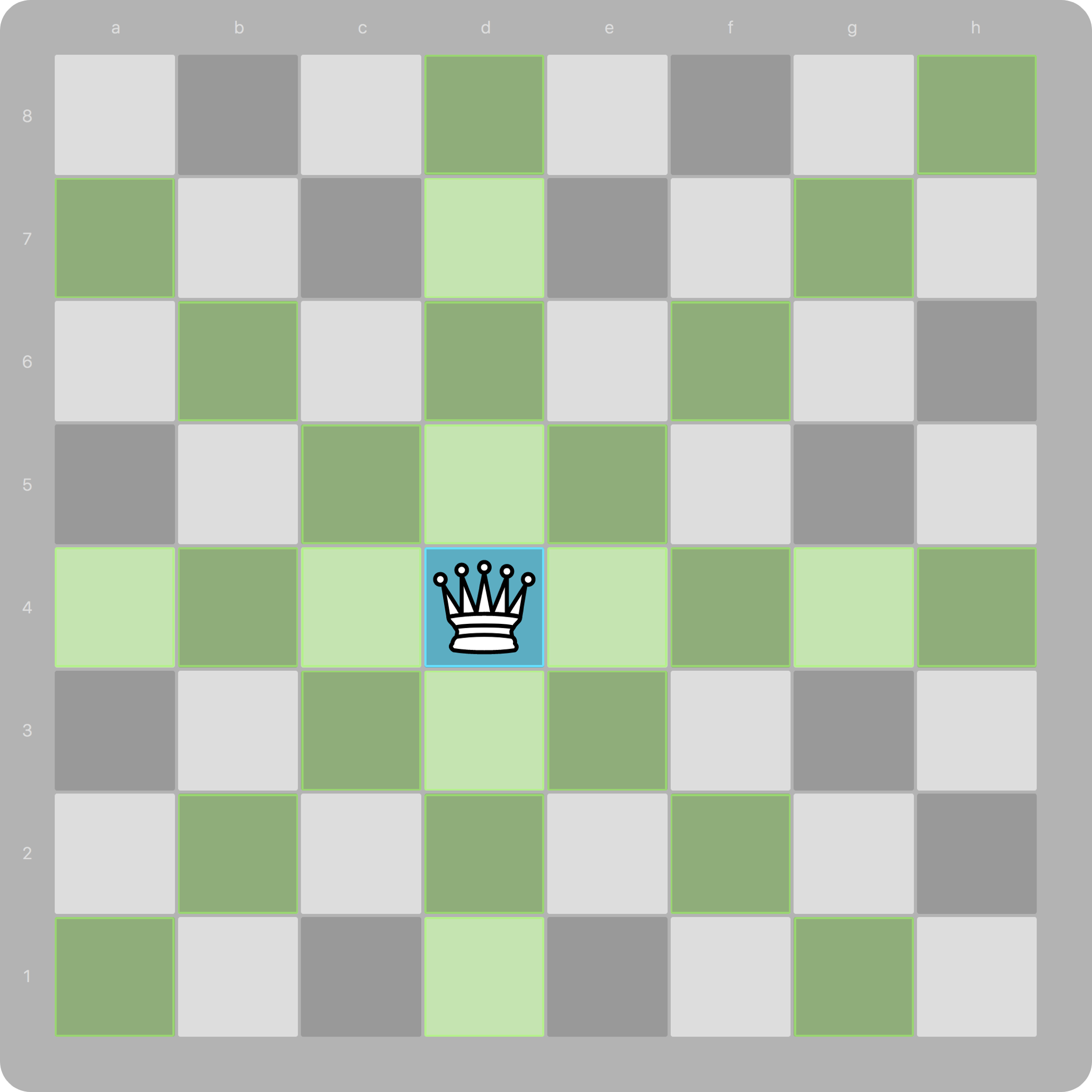That’s all for now, next time we’ll tackle the gnarly moves of the knight!

If you’re impatient for more, you can always check out the source code on GitHub at https://github.com/danbee/chess.

This post was also published to the thoughtbot blog.